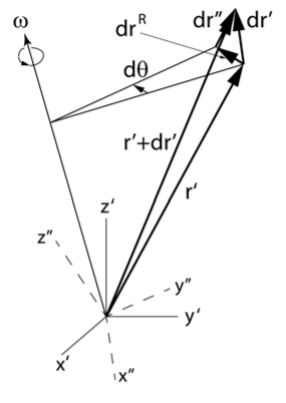# 13.3: Rigid-body Rotation about a Body-Fixed Point

$$\newcommand{\vecs}{\overset { \rightharpoonup} {\mathbf{#1}} }$$ $$\newcommand{\vecd}{\overset{-\!-\!\rightharpoonup}{\vphantom{a}\smash {#1}}}$$$$\newcommand{\id}{\mathrm{id}}$$ $$\newcommand{\Span}{\mathrm{span}}$$ $$\newcommand{\kernel}{\mathrm{null}\,}$$ $$\newcommand{\range}{\mathrm{range}\,}$$ $$\newcommand{\RealPart}{\mathrm{Re}}$$ $$\newcommand{\ImaginaryPart}{\mathrm{Im}}$$ $$\newcommand{\Argument}{\mathrm{Arg}}$$ $$\newcommand{\norm}{\| #1 \|}$$ $$\newcommand{\inner}{\langle #1, #2 \rangle}$$ $$\newcommand{\Span}{\mathrm{span}}$$ $$\newcommand{\id}{\mathrm{id}}$$ $$\newcommand{\Span}{\mathrm{span}}$$ $$\newcommand{\kernel}{\mathrm{null}\,}$$ $$\newcommand{\range}{\mathrm{range}\,}$$ $$\newcommand{\RealPart}{\mathrm{Re}}$$ $$\newcommand{\ImaginaryPart}{\mathrm{Im}}$$ $$\newcommand{\Argument}{\mathrm{Arg}}$$ $$\newcommand{\norm}{\| #1 \|}$$ $$\newcommand{\inner}{\langle #1, #2 \rangle}$$ $$\newcommand{\Span}{\mathrm{span}}$$$$\newcommand{\AA}{\unicode[.8,0]{x212B}}$$

With respect to some point $$O$$ fixed in the body coordinate system, the angular momentum of the body $$\alpha$$ is given by

$\mathbf{L} = \sum^{n}_i \mathbf{L}_i = \sum^n_i \mathbf{r}_i \times \mathbf{p}_i$

There are two especially convenient choices for the fixed point $$O$$. If no point in the body is fixed with respect to an inertial coordinate system, then it is best to choose $$O$$ as the center of mass. If one point of the body is fixed with respect to a fixed inertial coordinate system, such as a point on the ground where a child’s spinning top touches, then it is best to choose this stationary point as the body-fixed point $$O$$.Figure $$\PageIndex{1}$$: Infinitessimal displacement $$dr^{\prime}$$ in the primed frame, broken into a part $$dr^R$$ due to rotation of the primed frame plus a part $$dr^{\prime\prime}$$ due to displacement with respect to this rotating frame.

Consider a rigid body composed of $$N$$ particles of mass $$m_{\alpha}$$ where $$\alpha = 1, 2, 3, \dots N$$. As discussed in chapter $$12.4$$, if the body rotates with an instantaneous angular velocity $$\boldsymbol{\omega}$$ about some fixed point, with respect to the body-fixed coordinate system, and this point has an instantaneous translational velocity $$\mathbf{V}$$ with respect to the fixed (inertial) coordinate system, see Figure $$\PageIndex{1}$$, then the instantaneous velocity $$\mathbf{v}_{\alpha}$$ of the $$\alpha^{th}$$ particle in the fixed frame of reference is given by

$\mathbf{v}_{\alpha} = \mathbf{V} + \mathbf{v}^{\prime\prime}_{\alpha} + \boldsymbol{\omega} \times \mathbf{r}^{\prime}_{\alpha}$

However, for a rigid body, the velocity of a body-fixed point with respect to the body is zero, that is $$\mathbf{v}^{\prime\prime}_{\alpha} = 0$$, thus

$\mathbf{v}_{\alpha} = \mathbf{V} + \boldsymbol{\omega} \times \mathbf{r}^{\prime}_{ \alpha}$

Consider the translational velocity of the body-fixed point $$O$$ to be zero, i.e. $$\mathbf{V} = 0$$ and let $$\mathbf{R} = 0$$, then $$\mathbf{r}_{\alpha} = \mathbf{r}^{\prime}_{\alpha}$$. These assumptions allow the linear momentum of the particle $$\alpha$$ to be written as

$\mathbf{p}_{\alpha} = m_{\alpha} \mathbf{v}_{\alpha} = m_{\alpha} \boldsymbol{\omega} \times \mathbf{ r}_{\alpha}$

Therefore

$\mathbf{L} = \sum^N_{\alpha} \mathbf{r}_{\alpha} \times \mathbf{ p}_{\alpha} = \sum^N_{\alpha} m_{\alpha} \mathbf{r}_{\alpha} \times (\boldsymbol{\omega} \times\mathbf{ r}\alpha )$

Using the vector identity

$\mathbf{A} \times (\mathbf{B} \times \mathbf{ A}) = A^2\mathbf{B} − \mathbf{A} (\mathbf{A} \cdot \mathbf{B}) \notag$

$\mathbf{L} = \sum^{N}_{\alpha} m_{\alpha} [r^2_{\alpha} \boldsymbol{\omega} − \mathbf{r}_{\alpha} (\mathbf{r}_{\alpha} \cdot \boldsymbol{\omega}) ]$

The angular momentum can be expressed in terms of components of $$\boldsymbol{\omega}$$ and $$\mathbf{r}^{\prime}_{\alpha}$$ relative to the body-fixed frame. The following formulae can be written more compactly if $$\mathbf{r}_{\alpha} = (x_{\alpha }, y_{\alpha} , z_{\alpha} )$$, in the rotating body-fixed frame, is written in the form $$\mathbf{r}_{\alpha} = (x_{\alpha ,1}, y_{\alpha ,2} , z_{\alpha ,3} )$$ where the axes are defined by the numbers $$1, 2, 3$$ rather than $$x, y, z$$. In this notation, the angular momentum is written in component form as

$L_{i}=\sum_{\alpha}^{N} m_{\alpha}\left[\omega_{i} \sum_{k} x_{\alpha, k}^{2}-x_{\alpha, i}\left(\sum_{j} x_{\alpha, j} \omega_{j}\right)\right] \label{13.9}$

Assume the Kronecker delta relation

$\omega_i = \sum^3_j \omega_j \delta_{ij} \label{13.10}$

where

\begin{aligned} \delta_{ij} & = & 1 && i=j \\ \delta_{ij} & = & 0 && i \neq j \end{aligned}

Substitute \ref{13.10} in \ref{13.9} gives

\begin{align} L_{i} &=\sum_{\alpha}^{N} m_{\alpha} \sum_{j}\left[\omega_{j} \delta_{i j} \sum_{k} x_{\alpha, k}^{2}-\omega_{j} x_{\alpha, i} x_{\alpha, j}\right] \notag \\ &=\sum_{j}^{3} \omega_{j}\left[\sum_{\alpha}^{N} m_{\alpha}\left(\delta_{i j} \sum_{k} x_{\alpha, k}^{2}-x_{\alpha, i} x_{\alpha, j}\right)\right] \end{align}

This page titled 13.3: Rigid-body Rotation about a Body-Fixed Point is shared under a CC BY-NC-SA 4.0 license and was authored, remixed, and/or curated by Douglas Cline via source content that was edited to the style and standards of the LibreTexts platform; a detailed edit history is available upon request.